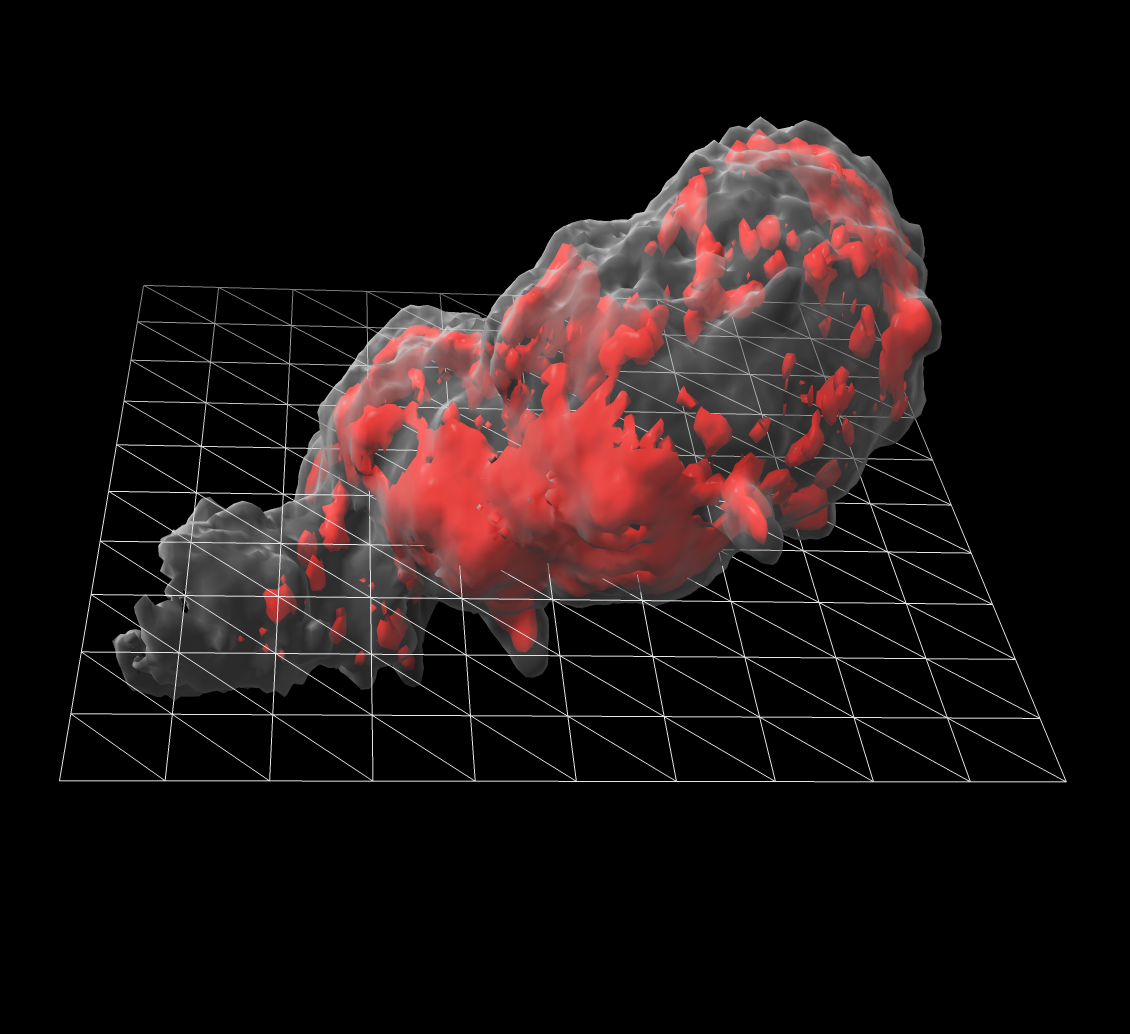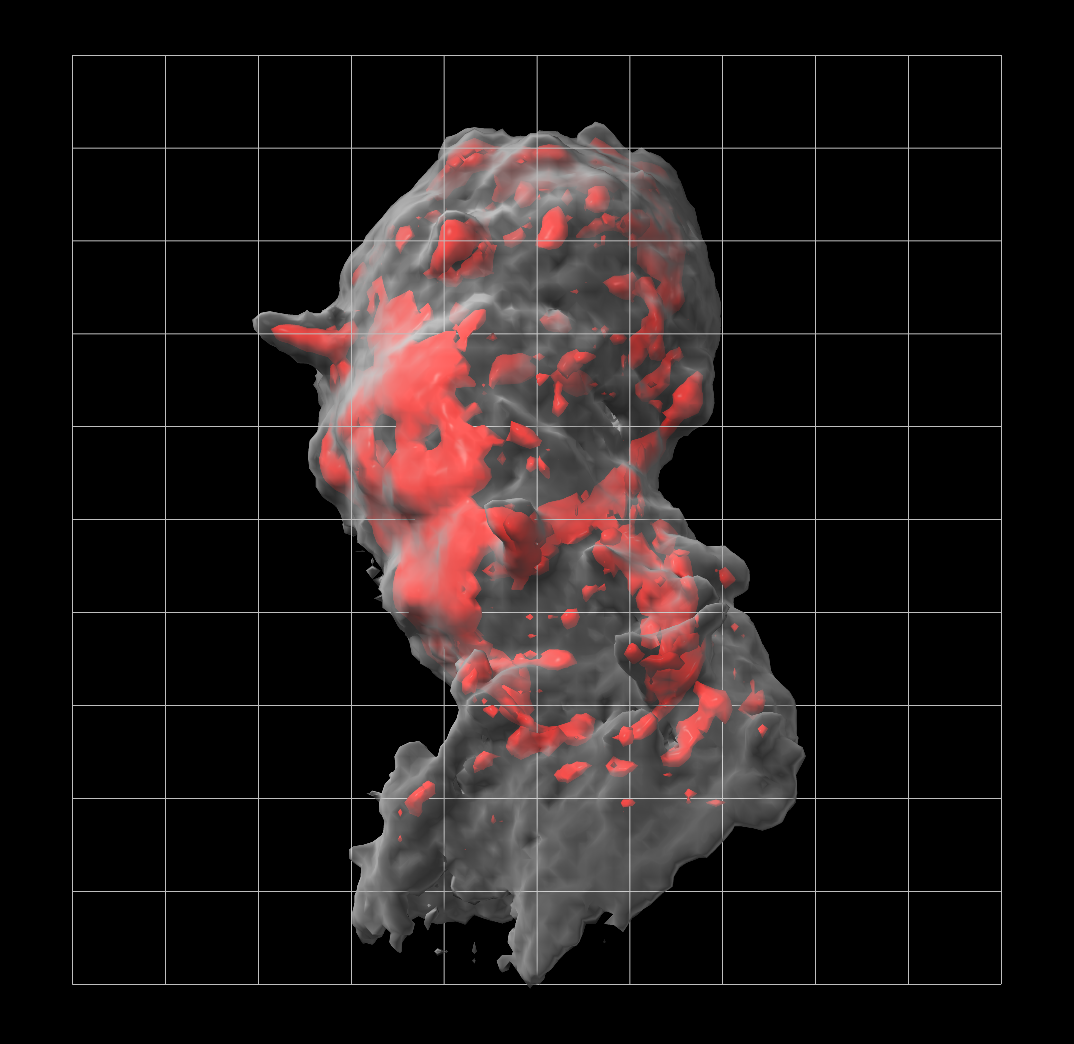# Hide diagonal lines of a mesh surface

Here is some ChimeraX Python code that hides lines of a mesh that are not parallel the x or y or z axes. Ilhan Tomris asked about how to show a grid on light microscopy data. The shape command can show a rectangle, and the surface style command can display it as a mesh.

`````` shape rectangle width 20 height 20
surface style #3 mesh
``````

But the rectangle is made up of triangles so the mesh shows triangles. The Python code hide_diagonals.py below defines a command hidediagonals that hides the lines that are not parallel x or y or z axes.I also added this to ChimeraX as the surface squaremesh command.

```# Define command hidediagonals that hides edges of a mesh surface that are not parallel
# the x, or y, or z axes.  This was to make a square grid to overlay on microscopy data.

def hide_diagonals(session, surface):
vertices = surface.vertices
from numpy import array, uint8

'''
The edge mask uses the lowest 3 bits to indicate which of the 3 edges
of a triangles should be shown.
'''
i0,i1,i2 = triangle
v0,v1,v2 = vertices[i0],vertices[i1],vertices[i2]
if parallel_x_or_y_or_z(v0,v1):
if parallel_x_or_y_or_z(v1,v2):
if parallel_x_or_y_or_z(v2,v0):

def parallel_x_or_y_or_z(xyz1,xyz2):
return (xyz1 == xyz2).sum() >= 2

def register_hide_diagonals_command(logger):
from chimerax.core.commands import CmdDesc, register, SurfaceArg
desc = CmdDesc(
required = [('surface', SurfaceArg)],
synopsis = 'Hide diagonal of surface mesh'
)
register('hidediagonals', desc, hide_diagonals, logger=logger)

register_hide_diagonals_command(session.logger)

```

Tom Goddard, October 18, 2020